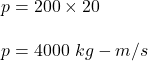## What is the momentum of a 200 kg truck travelling at 20 m/s?

Question

What is the momentum of a 200 kg truck travelling at 20 m/s?

in progress 0
1 month 2021-08-31T14:08:38+00:00 1 Answers 0 views 0

p = 4000 kg-m/s

Explanation:

Given that,

The mass of a truck, m = 200 kg

Speed of the truck, v = 20 m/s

We need to find the momentum of the truck. The formula for momentum is given by :

p = mv

so,So, the momentum of the truck is equal to 4000 kg-m/s.